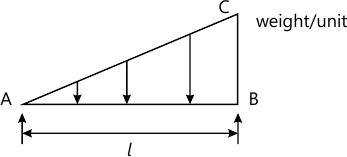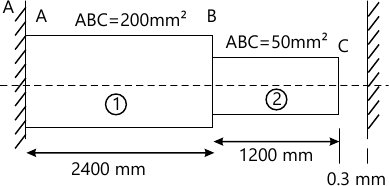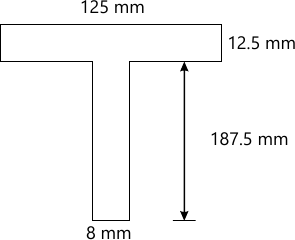MORE IN Strength of Materials
MU Civil Engineering (Semester 3)
Strength of Materials
May 2014
Total marks: --
Total time: --
INSTRUCTIONS
(1) Assume appropriate data and state your reasons
(2) Marks are given to the right of every question
(3) Draw neat diagrams wherever necessary

1 (a) Derive relation among bending moment, shear force and rate of loading.
5 M
1 (b) What is modulus of Elasticity. Bulk modulus and modulus of Rigidity state Relation between them.
5 M
1 (c) State assumptions made in therory of simple Bending.
5 M
1 (d) Find maximum shear stress induced in a solid circular shaft of diameter 150mm. 180Rpm.
5 M

2 (a) Sketch SFD and BMD for beam shown in fig10 M
2 (b) Determine the support reaction at A and C when AB is loaded axialy by 7..at B as shown in fig. True E=200×103Mpa.10 M

3 (a) Two wooden planks 150mm × 50mm each are connect to from at section of a beam if a moment of 3.4kn.m is applied around a horizontal neutral axis, inducing tension below the neutral axis, Find stresses at the extreme fibers of the cross section. Also calculate total tensile force on cross section.
10 M
3 (b) A bar 1.4cm in diameter gets stretched by 0.25cm under a steady load of 8kn. What stress would be produced in same bar by a weight of 0.8kn. Which falls freely vertically through a distance of 7cm to a rigid collar attached at its end .
Take E=200 Gn/m2.
10 M

4 (a) A T beam of span 5m bas a flangel 12.5mm × 125mm and web 187.5 mm × 8mmm. If maxm permissible stress is 150mpa. Find maximum u.d.l the beam can carry.10 M
4 (b) A shaft rotating at 150rpm. And transmits a power of 300kw. The diameter of the shaft is 100 mm. Maximum torque is 25% more than the mean torque what is the Magnitude of torsional shear stress and twist 9 length of shaft is What is the Magnitude of torsional shear and twist 9 length of shaft is 1.5m G=85 Gpa.
10 M

5 (a) A square column of 400 mm × 400 mm size is subjected to an axial load of 400 kn. In addition to this a load of 40kn. Is acting at an eccentricity of 20mm about x-x and y-y axes. Find stress at all four corners.
10 M
5 (b) A follow cast iron column of 200mm external diameter 100mm internal diameter and 8m Long has both end fixed. If is subjected to axial compressive load. Taking factor of safety as 5.6c=540N/mm2
$\alpha =\dfrac{1}{1600}$ determine safe Rangine load.
10 M

6 (a) A cylindrical shell , 3m long is having 1m internal diameter and 15 mm thickness. Calculate hoop stress. Longitudinal stress, maximum intensity of shear stress and change in diameter of shell if is subjected to an internal fluid pressure of 1.5Mpa.
Take E=200 Gpa and poison's ration=0.3
10 M
6 (b) Two actually perpendicular planes of on elements of material are subjected to tensile stress of 100N/mm2. Compressive stress 40N/mm2 and shear stress 60 N/mm2 and shear stress 60 N/mm2. Find
i) Magnitude and direction of principle stressed.
ii) Magnitude of normal and shear stress on a plane on which shear stress is maximum.
10 M

More question papers from Strength of Materials